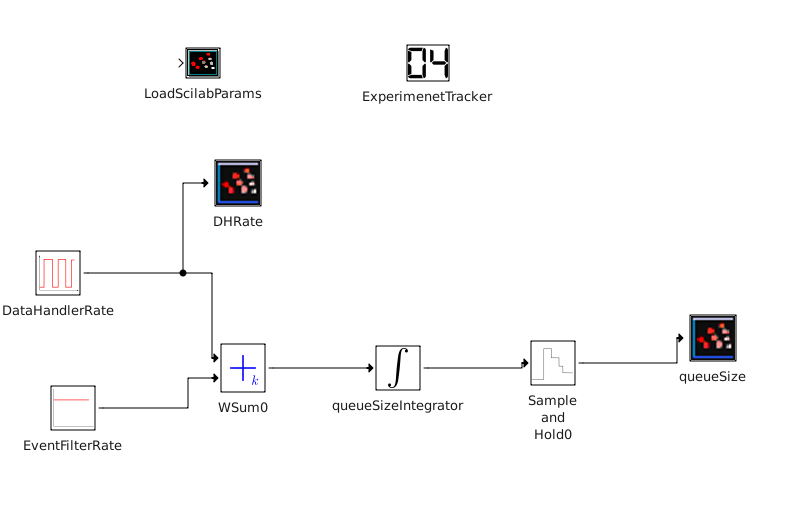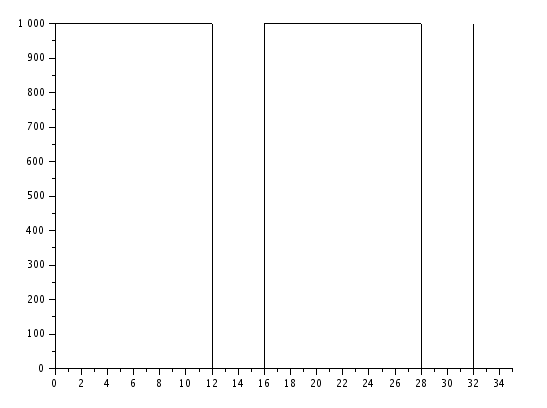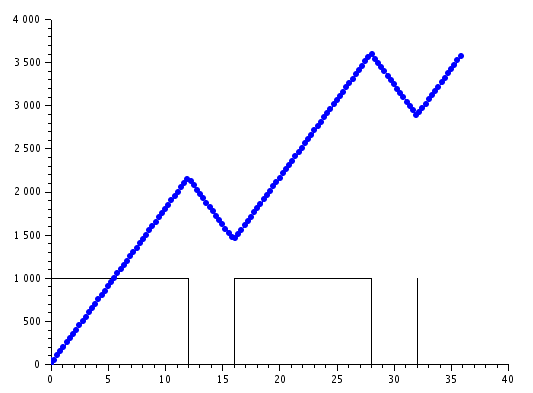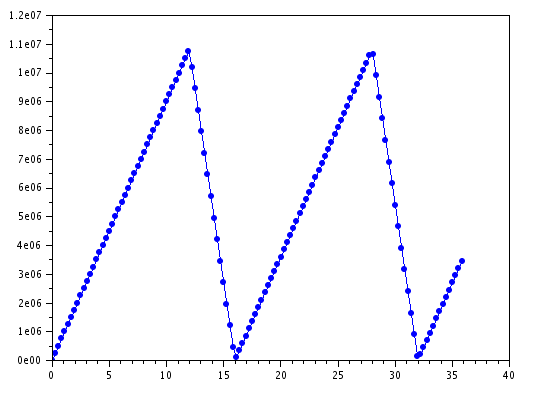TWiki>Main Web>MatiasAlejandroBonaventura>TDAQSimulation>PowerDEVSProyect>PowerDEVSGettingStarted>HowToContinuosModel (2016-05-04, RodrigoDanielCastro)

# How to model a simple continuous dynamic system in PowerDEVS

In this guide we are going to learn how to use the basic PowerDEVS features to model a very simple representation of the TDAQ system for Phase II, using a continuos blocks.

It assumes you already have installed and configured PowerDEVS correcty, and that you read and have a good background of DEVS theory.

We will model the same system as in the PowerDEVSGettingStarted, but in this case focusing only on the average values of the DataHandler, StorageHandler, and EventFilter. You will see that the simulation time is almost instantaneos as very few calculations are needed.

In PowerDEVSGettingStarted a discrete model is developed. In this guide we will develop a continuos model focusing on the average queue size.

## First things first - What will we model?

We will model the same system as in the getting started guide (a simple abstraction of the StorageHandler for the TDAQ PhaseII): You can read about this system here.

In PowerDEVSGettingStarted a discrete model was developed, taking into account arrival distributions and job sizes, etc. Nevertheless, if we were only concerned about the queueSize for the average rates of the StorageHandler and EventFilter, we can solve it in an analitical manner.

If we suppose that the DataHandler generates events a a rate DH and the EventFilter processes events at rate EF, then the queue size Q will fill at DH-EF.
Because we what to know the queueSize after certain time, it is better to express the queueSize in term of the time advance:NOTE: This would read: the speed at which the queueSize Q changes respect to time t (), is equal to the difference between the input rate minus the output rate (DH - EF).

So, in this guide we will model this equation (1).

NOTE: This is a very simple system (only one single linear equation). In PowerDEVS you can model systems of non-linear equations, and you can find examples of this in the /examples/continues folder of PowerDEVS (eg lotka_volterra).

## Step 1 - First Simulation

### Model - represent the equation in PowerDEVS

Model setup

In this section we will add a single atomic model and run our first simulation.

1. For this guide we will use the getting_started branch in GIT repository: git checkout -b getting_started
2. Open PowerDEVS simulator (it will also open Scilab)
3. File -> New, to create a new top model
4. Add the following models (drag&drop from library):
1. ScilabCommand (in the Sinks lbrary). Name it LoadScilabParams.
2. ExperimentTracker (in the Queueing Networks library). Name it ExperimentTracker.

These models provide infrastructure to read parameters, so parameter sweeping, interaction with scilab, etc. One day a bright student will add this functionality to the core of PowerDEVS :-)

5. File--> Save, to save the model. Save the models under /examples/<yourUserName>/MyGettingStarted/ContinuosGettingStarted.pdm when requested.
NOTE: all top models should be stored under the /examples folder. It is recommended that each user creates its own folder for its custom models, and a folder for each model. They can be placed diferently if desired.
6. Before running the simulation, we must take care of the parameters.
There are several ways of setting the parameters for PowerDEVS atomic models. The easiest and straight forward way is to double-click in the atomic model and set the values for each of the parameters. Although this seems easy, it does not scale if you have several atomic models (imagine double-clicking 100 models to see the parameters!). So we will see here how to specify parameters in a separate Scilab file (hopefully a good student will make this steps automatic in the future):

1. In the folder where you saved the top model (ej /examples/<yourUserName>/MyGettingStarted/) create a new folder called Sciab
2. In the new Scilab folder create a new file called model.scilabParams.
3. Now we need to configure things in order for this file to be loaded by Scilab. Double-click the LoadScilabParams model, and in the "Run at Init" parameter put the following: exec('../examples/<yourUserName>/MyGettingStarted/model.scilabParams', 0). This will make the file you created before to be loaded in Scilab when the simulations first starts.
4. Click the Priority button in PowerDEVS GUI. This menu allows you to specify the Z function (which will decide the priority of execution of each model). Make sure that LoadScilabParams, ExperimentTracker and UpdateScilabParams are the first 3 models. FinalizationCommands should be always the very last model. (Probably for this guide now, you will have to change only the FinalizationCommands model. But whenever you add a new model, remember to check this).
5. This file will be loaded by Scilab so you can put any valid Scilab expression here (constants, variables, math expression, even complex functions).
Creating the model
1. Add a new QSSIntegrator model (drag&drop from the Continuos library). Name it queueSizeIntegrator.
This model will represent the current size of the queue. This model will do the integration calculations (using the QSS integration method), as the queueSize is express as a derivative in equation (1). The input for the integrator will be the derivate of Q () and the output will be the current value Q.

So, how do we calculate the derivate of Q ()? Easy, we look at the formula (1). It is DH-EF. Lets add that to PowerDEVS.

2. Add a new Constant model (drag&drop from the Sources library). Name it DataHandlerRate.
Double click on the model and set the parameter K to DataHandler.rate.
3. Add a new Constant model (drag&drop from the Sources library). Name it EventFilterRate.
Double click on the model and set the parameter K to EventFilter.rate.
This models will represent the DH and EF rates. For now, this rate are constant as they don't change along with time. The value for the rates will be taken from the parameters file model.scilabParams (created at the begging, and we will add values at the end)

4. Acording to (1) we should substract these values (DF - EH). Add a new WSum model (drag&drop from the Continuos library).
The WSum model implements the addition of multiple signals, according to the QSS method.

5. Connect the DataHandlerRate output to the first input of the WSum
6. Connect the EventFilterRate output to the second input of the WSum
7. Double-click the WSum model and set the second parameter to -1.
Note the formula in the description of the model. This way we are configuring the model to substract the second input (now connected to EF) to the first one (now connected to DF), so the output will actually be DF-EF.

8. Connect the WSum output to the input of the queueSizeIntegrator.
So here we say that the output of WSum, which equals DF-EF,is the input for the integrator. And by (1) we know that. So the output of the integrator is the queueSize Q.

This model represents formula (1)

Parameters and output

Before we can start the simulation, we should take care of the parameters ( DataHandler.rate and EventFilter.rate). Also we will add a model to track the output of the simulation in Scilab.
Also, the default QSS method is an order 3 integration method which solves linar ecuation in one single step. To be able to see the output as expected we will add a model which will hold the integrator results and sample in regular period. Another option would be changing the integration method to QSS1 which will need more integration steps.

1. In the model.scilabParams file that you creted at the beggining, add the following lines to set values for the parameters. Lets set the EventFilter to process half of what the DataHandler produces:

DataHandler.rate = 1000;
EventFilter.rate = 500;
ParameterValues =  ;

NOTE: the ParameterValues is used to swap parameters and explained in the HowToSwapParametersHowTo . In the future, some good student will remove the need of declaring it if not needed.
2. Add a new Sample and Hold model (drag&drop from the Hybrid library).
3. Connect the output of the queueSizeIntegrator model to the input of the Sample and Hold model. Double-click the model to set the T Sampling parameter to 100
4. Add a new doubleValueSampler model. This one is programmed but ont in the library GUI. To use it:
1. Add a new Atomic model (drag&drop from the Basic Elements library).
2. Right-click --> Edit --> Code
3. From the GettingStarted folder, chooose the doubleValueSampler.h file.
4. Click OK.
5. Name the new model queueSize. Double-click to set the SamplerPeriod parameter to -1.
6. Connect the output of the Sample and Hold model to the input of the queueSize model.

### Simulate

1. Now we are ready to simulate. Click the "Simulate" button [ADD icon] and you will see the Simulation GUI.
2. In Final Time choose how many seconds you want to simulate (this is virtual simulation time, not real wall clock time). This should simulate really quickly, so lets simulate 36hours = 129600 seconds
3. Click Run Simulation. You will see thebar below showing progress. Probably for this small simulation it will finish really fast. The the simulation finishes in the bar below you will see "Simulation Completed xxxx ms" with the real wall clock time the simulation took to finish (mine took 123ms).

Note that for this very simple model, the execution time of the simulation only depends on the amount of samples we want to get (currently one every 1000s). The calculation of results is done in one single step, independently of the time we want to simulate, and the parameters (the rates) we choose.
In contrast with the discrete simulation, here we are not generating job one by one, so the rates of the EventFilter and DataHandler don't affect the simulation execution time. More over, because the equation is linear QSS3 solves it in one single integration step, so the simulation execution time is not affected by how many seconds we want to simulate.

### Analysing simulation results in Scilab

There many many ways to see and analyze PowerDEVS simulation results. You can use Python, CSV, Scilab, Mathlab, etc. Usually we use R as it has several interesting functions and plots, sometimes we upload plots to plot.ly. When we need to debug or see the behaviour of models in great great details we use the debug log, which PowerDEVS puts in ouput/pdevs.log.

1. Switch to the Scilab GUI. You will notice that in the main console there are some messages about the simulation starting and finished.
2. Type who in the main console all the available variables will be listed. Also the top right panel (Variable Browser) will update showing the variables.
3. Type: scf(); plot(queueSize_t, queueSize_max, ".-")## Step 2 - Adding inactivity times for the DataHandler

Lets complicate the model a bit: The ATLAS experiment will run in cycles of 16 hours. In those 16 hours, the LHC will have collisions during 12 hours, then the beam is dumped and the LHC takes about 4 hours to accelerate and collide the new beam. So, in our model that means that the DataHandler will send data during 12 hours, but then during 4 hours it will stop its activity. Nevertheless, events are stored in the StorageHandler so the EventFilter can continue processing during those 4 hours.
How should be change the model to accommodate this change?

With most usual integration methods ( Euler, Runge-Kutta, etc) which do time discretization it is not trivial to introduce discontinuities, as if the discontinuity falls in the middle of a discretization step the method has to go a step back and do recalculations. For QSS, as it is implemented with discrete events, the discontinuity is just another event and is treated transparently.

TODO: En este caso como la discontinuidad es temporal, no se si vale lo que pongo arriba. Para los metodos clasicos les cuesta cuando el sistema cambia su dinamica en base al valor de sus variables. Aca cambia la dinamica con el tiempo, asique no se si aplica.

### Model - changing constants

So now the DataHandlerRate is not constant in time any more. It will keep the same value for 12 hours and the be 0 for 4 hours. To model this, we will use the Square model that sends a square signal with the configured amplitud and frequency.

1. Delete the DataHandlerRate model.
2. Add a new Square model (drag&drop from the Sources library). Name it DataHandlerRate.
1. Double click on the model and set the parameter a to DataHandler.amplitud
2. Double click on the model and set the parameter f to DataHandler.frequency
3. Double click on the model and set the parameter d to DataHandler.dutyPercentage
4. Double click on the model and set the parameter start to DataHandler.start (if you fon't see this parameter add it manually from Edit-->Parameters)
3. Connect the output of DataHandlerRate model to the input of the WSum model.
4. Lets add the parameter values. In the model.scilabParams file that you creted at the begging, add the following lines to set values for the parameters.

DataHandler.amplitud = 1000;
DataHandler.frequency = 1/57600;
DataHandler.dutyPercentage = 75
DataHandler.start = 1

We are setting the amplitud to 1000, so that when it is working it will generate events at 1000 KHz. The frequency to 1/57600= one cycle every 16 hours. The duty is 75%, 12 hours with high amplitud, 4 hours without generating. The start parameter determines if the first signal should be at the low or high amplitud.

5. Lets add another sampler so that we can track the rate at which the DataHandler generates events. Add a new doubleValueSampler model (you can copy&past from the queueSize sampler now).
Name it DHRate and connect it to the output of the DataHandlerRate### Analysing simulation changes

1. Simulate, as in the previous steps. Run for 129600 seconds, 36 hours, more than 2 cycles of activity-inactivity
2. Plot the DataHandlerRate in Scilab: scf(); plot2d2(DHRate_t / 3600, DHRate_max, 1)
Note that we are plotting 'DHRate_t / 3600', so that we see time in hours instead of seconds. We use plot2d2 to see the function as stairs (scilab by default interpolates points which would not be approapiate here).Verify that the DataHandler is generating evets at 1000kHz during 12 hours, then it stops generating and stays idle for 4 hours (from 12 to 16), and then starts again.

3. Lets plot the queue lenght again now: plot(queueSize_t / 3600, queueSize_max / (1000 * 10), ".-")
Here we also plot time in hours, and we normalize the queue size so that we can see both functions in the same plot.As you can see, while the DataHandler is generating events, the amount of queued jobs in the DataStorage grows linearly. When the DH stops generating jobs, the EF keeps consumig, so the stored jobs decreases.
Nevertheless, we can see that the computing power we configured for the EF (500 kHz events) is not enough to process all the events during one cycle (the second cycle starts with 15M events in the SH)

4. You could set the EventFilter.rate parameter as follows in order to make sure storage is completely empty at the start of each cycle: EventFilter.rate = DataHandler.rate * (DataHandler.dutyPercentage/100);## Step 3 - Not allowing storage to go below cero

If we configure that the high values for the computing power of the EF, with this model the SH queue can go below cero (we never modeled that queues can be negative).

You could set the EventFilter.rate parameter as follows in order to make sure storage is completely empty at the start of each cycle: EventFilter.rate = DataHandler.rate * (DataHandler.dutyPercentage/100);

TBC: use the CrossDetect model to model this dicontinuity in the model.

-- MatiasAlejandroBonaventura - 2016-04-29

Topic attachments
I Attachment History Action Size Date Who Commentpng Selection_068.png r1 manage 7.3 K 2016-04-29 - 19:09 MatiasAlejandroBonaventura
Topic revision: r4 - 2016-05-04 - RodrigoDanielCastroLog In

Webs

Welcome GuestCern SearchTWiki SearchGoogle Search Main All websCopyright &© 2008-2020 by the contributing authors. All material on this collaboration platform is the property of the contributing authors.
Ideas, requests, problems regarding TWiki? Send feedback